# Measurement of Electrical Energy

Electrical energy is the product of electrical power and time, and it is measured in joules. It is defined as “1 joule of energy is equal to 1 watt of power is consumed for 1 second’’.
i.e.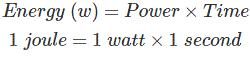Energy and power are closely related. Electrical energy can be measured only when electrical power is known. So first, we understand electrical power. Electrical power is the amount of electrical current that results from a certain amount of voltage or we can say that power is the rate at which energy is delivered. It is measured in watts. Mathematically it is written as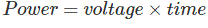The measurement of electrical energy is completely dependent on power which is measured in watt, kilowatts, Megawatts, gigawatts, and time which is measured in an hour. Joule is the smallest unit of energy. But for some bigger calculation, some better unit is required. So, the unit used for electrical energy is watt-hour.
Watts are the basic unit of power in which electrical power is measured or we can say that rate at which electric current is being used at a particular moment.

## Units of Electrical Energy

• Watt-hour is the standard used for measurement of energy, describing the amount of watts used over time. It shows how fast the power is consumed in the period of time.
•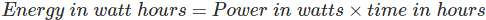• Kilowatt-hour is simply a bigger unit of energy when large appliances drawn power in kilowatts. It can be described as one kilowatt hour is the amount of energy drawn by the 1000 watts appliance when used for an hour.Where, One kilowatt = 1000 watts
•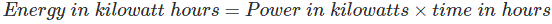• Megawatt-hour is the unit of energy which normally used when output of power generation is very large. In power plant,’ Megawatt’ term is used to show the power generation capacity of power plant.
•Solved Example for Showing Calculation
Problem – A consumer uses a 8 KW geezer, a 5 KW electric press and four 100 watt bulbs for 10 hours. How many units (KWh) of electrical energy have been used.
Solution –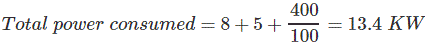Time taken = 10 hours
Therefore,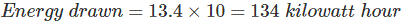Want To Learn Faster? 🎓
Get electrical articles delivered to your inbox every week.
No credit card required—it’s 100% free.